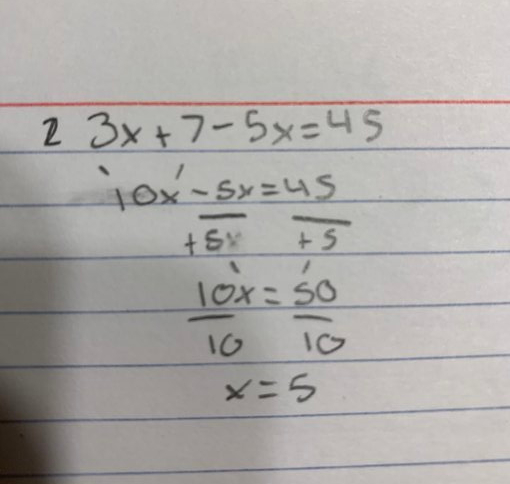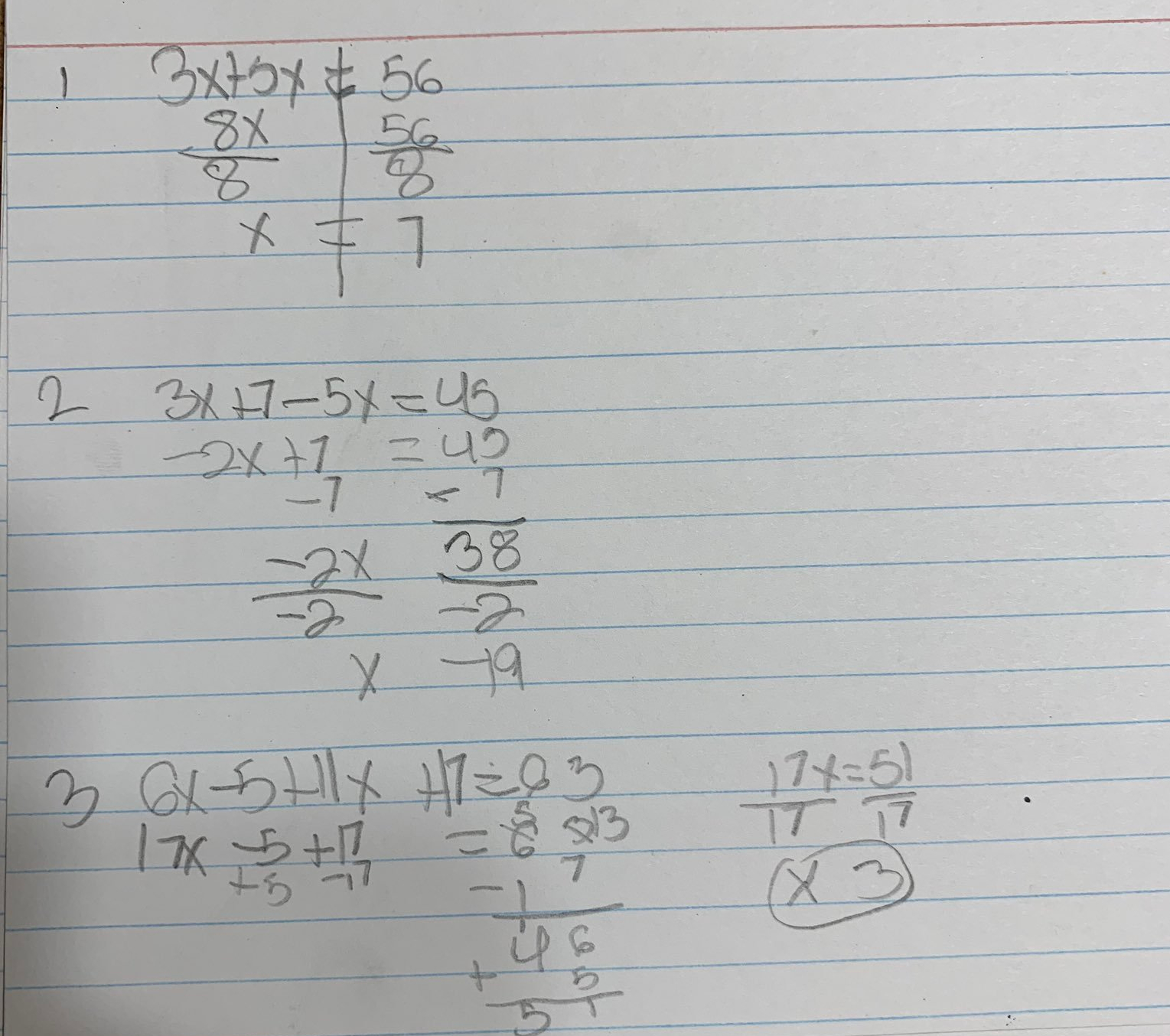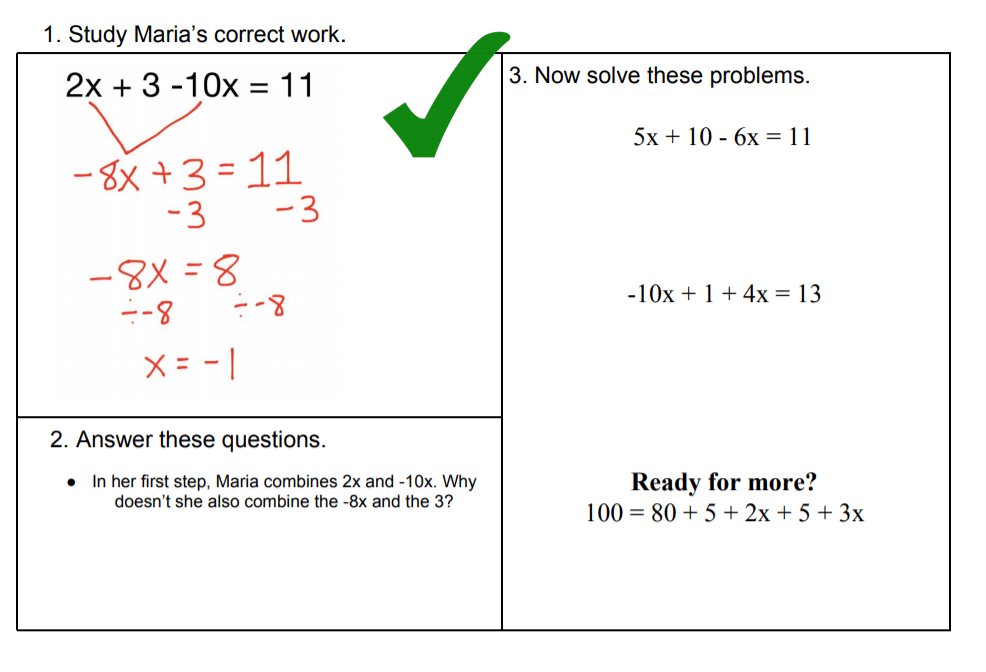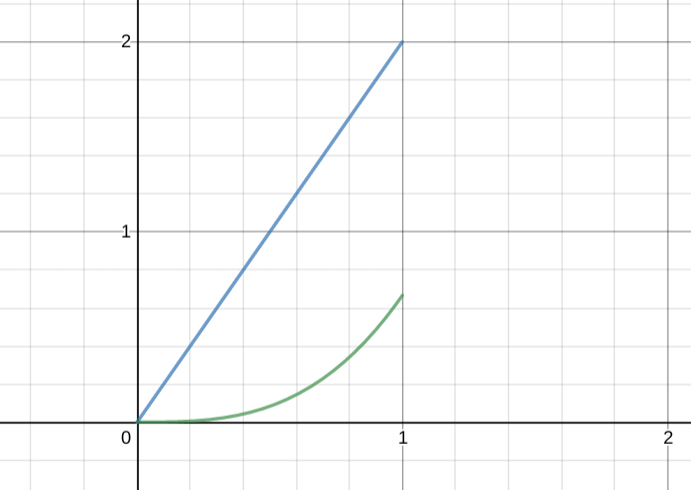## Dear Aunt Sally: The exit tickets are all over the place!

Dear Aunt Sally,

My students had a hard time with my lesson on solving equations. How do I know? I gave a short “exit ticket” and the results were…mixed. There were three questions:

• 3x + 5x = 56
• 3x + 7 – 5x = 45
• 6x – 5 + 11x + 17 = 63.

The main issue is combining like terms. (I’ve included some pictures of student work for you, Aunt Sally.)That said, some students in each class definitely did understand the material.One more issue. In most of my classes, students didn’t struggle with the first problem. But in each of my classes, some students did, and in one of my classes very few students got that first one correct either.

What do I do to help the students who didn’t understand the material, and what do I do with the kids who did?

Ryan in Florida

***

Dear Ryan,

You have described one of the perpetual struggles of teaching. How do I balance the needs of the many versus those of the few? I suppose back in Neanderthal times the fellow charged with teaching youth to spear a mammoth came home to the cave feeling similarly. “Some of those kids get it,” he’d say. “But what about the one who used the flat end of the spear? He’s going to starve to death. He would really benefit from getting back to the basics. Maybe I’ll split the group in half?”

Thank goodness we aren’t Neanderthals. We are modern teachers! This means we have access to the wonders of modern technology. I of course mean the blackboard and copy machine.

My favorite way to follow-up on these sorts of quizzes is with examples, so I put together three such activities for you:And then some mixed-up practice, to take another step in the right direction.A good example activity, in my experience, can seem so simple so as to hide its design. And in fact the actual design of the student work was mostly straightforward. The choices to make are mostly ones I made long ago — to prefer simplicity, to subtly use arrows and lines, and to make the work as much like a student’s as possible while making the work as clear as possible to read.

(The mistake, in particular, closely follows the design of an Algebra by Example mistake.)

Most of the work here is in narrowing in on a specific type of problem that is worth including in the examples. (The simplicity of the format works well with the more complex task of narrowing in.)

Here is a rule I tell myself while looking at a student’s mistake: Every mistake points to a family of situations very similar to the mistake that the student doesn’t yet know how to handle. Find that family, and teach it!

In this case I don’t assume the mistakes on the second problem (3x + 7 – 5x = 45) point not to issues “combining like terms” in general. I assume instead that the mistake points to issues where the like terms are separated visually in the equation and where subtraction is involved. Those things are distinctive and make the equation more difficult to solve correctly — that is the family that I focus in on for the example.

How the examples are used is your decision, Ryan, but here is how I would do it. Begin class by showing just the example, and ask students to silently study it. (They can let you know when they’ve read it all with their thumbs.) When finished, you can ask them to answer the explanation questions on their own or with a partner. If you want to interject with an explanation, by all means, but often students are ready to dive straight into the practice problems.

There are three examples/non-examples I’ve made. Use as many as your class would benefit from.

Then, there are four practice problems. Those are important because they are mixed practice. If we see each of these examples as pointing to a micro-skill, a small family of problems within the broad category of “two-step equations,” then these problems are interleaved in the practice set. That can be useful! Your students will have to think back and remember what they did with the examples.

Teachers of equation-solving know that there are always more mistakes that need to be addressed. The difference between good and bad teaching of this topic, as I see it, is whether the teacher can get specific and point to precisely what is hard for their students. Subtracting, different visual formats, handling a variable with coefficient 1 (x as 1x), and so many other little things — there will always be more of these. Some teachers just repeat and repeat and repeat without getting any more specific. Instruction should get more specific as practice gets more general.

So, keep at it! Pretty soon your young charges will be scattering the landscape with Woolly Mammoth carcasses, so to speak.

-Aunt Sally

## A Syntax of Geometrical Figures in “De Aetatibus Mundi Imagines”Holanda represents the intelligible reality of the Holy Trinity through a “hypothetical” syntax of geometrical figures:

“Starting from a perfect circle, three triangles merge in the abyss, provoking a strange sensation as much of movement as of immobility. Alpha and Omega are inscribed on the first equilateral triangle, perfectly inscribed in the circle.”

Spectacle and Topophilia by David R. Castillo

Bonus images:## Introducing Stable Distributions

The story so far:

• There are lots of ways to put two functions together and get a new one out of the process.
• Addition is one of these ways. Multiplication is another.
• If you add two Gaussian functions together, you don’t get another Gaussian function.
• If you multiply two Gaussian functions together, guess what? You get another Gaussian function.
• Convolution is another way of combining two functions.
• If you convolve two Gaussian functions together, guess what? You get another Gaussian function.

In the last post I tried to explain why convolving two probability distributions produces the distribution of the sum of those variables. And that sum is guaranteed to also be a bell curve, as long as the distributions its made of come are distributed normally as well.

It’s worth stewing for a moment on what that means, because a lot of things that we care about can be thought of as sums of random variables.

One example is height. Take, for example, the height of a forest. I know nothing about the biology of forest height, but I did find a few figures online. (Yes, random searching.)

Here they are.Some of these plots look normal. Then again, some of them don’t. I don’t really care! Let’s pretend that forest height truly is normally distributed. Why would that be?

The thing is that there are a lot of factors that go into how tall a forest is height. There is underlying genetic variation in the families of trees that make a forest. There is underlying randomness is the environmental conditions where a forest grows, and “environmental conditions” is itself not a single factor but another collection of random variables — rain, soil, etc.

If we were going to make a list of factors that go into forest height how long do you think it would be before we had an exhaustive list? Hundreds of factors? Thousands?

Given all this, “forest height” really isn’t a single random variable. It’s a collection of random variables, not the way we think of a single coin flip.

(AND IS A COIN FLIP EVEN REALLY A SINGLE RANDOM EVENT??? OOOOOOOH DID I BLOW YOUR MIND?)

This all feels very complicated. How is it that a forest’s height has such a lovely normal distribution?

Given the math in the previous post we have a very tidy answer: forest height is thought of as a sum of random variables. Even if there are hundreds or thousands of underlying random variables that sum up to forest height, as long as all (most?) of them are themselves Gaussian, so will their sum, represented by the convolution of all those thousands of distributions.

(Note: we did not prove that convolution preserves the Gaussian nature of a distribution for an arbitrary number of distributions. But if you take two of those distributions they make a new Gaussian, and then pair that with another distribution, and then another, etc., so on, it’s a pretty direct inductive argument that you can convolve as many of these as you’d like and still get a Gaussian at the end of things.)

So convolving is nice because it gives us a tidy way to think of why these incredibly complex things like “forest height” could have simple distributions.

But there’s only one problem: who says that you’re starting with a Gaussian distribution?

Convolution does not always preserve the nature of a function. To pick an example solely based on how easy it was for me to calculate, start with a very simple function:$f(x) = 2x$

Define this only on the region$[0,1]$ so that it really can be thought of as a probability distribution. Then, convolve it with itself.(Hey, why is this only the first half of the convolution? Because for the life of me I can’t figure out how to visualize the second half of this integral while limiting the domain of the original function. Please, check my work, I am actually pretty sure that I’m exposing an error in my thinking in this post but hopefully someone will help me out!)

In any event, what you get as a result of this convolution is certainly not linear. So linearity of a distribution is not preserved by convolution.

What this means is that whether convolution can explain the distribution of complicated random variables that are the sum of simpler random things really depends on what the distribution we’re dealing with is. We are lucky if the distribution is Gaussian, because then our tidy convolution explanation works. But if the distribution of the complicated random variable was linear (what sort of thing even could) then this would present a mystery to us.

But is it just Gaussian distributions that preserve their nature when summed? If so, this would be pretty limiting, because certainly not every distribution that naturally occurs is a normal distribution!

The answer is that it’s not just Gaussian distributions. There is a family of distributions that is closed under convolution, and they are called the stable distributions.

***

I started trying to understand all of this back when I tried to read a paper of Mandelbrot’s in the spring. Getting closer!

This post doesn’t follow any particular presentation of the ideas, but a few days ago I read the second chapter of Probability Tales and enjoyed it tremendously. There are some parts I didn’t understand but I’m excited to read more.